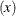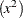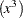# Shared Concepts and Topics

### Other Parameterizations

This section applies to the following procedures:CATMOD, GENMOD, GLMSELECT, LOGISTIC, PHREG, and SURVEYPHREG.

Some SAS/STAT procedures, including GENMOD, GLMSELECT, and LOGISTIC, support nonsingular parameterizations for classification effects. A variety of these nonsingular parameterizations are available. In most of these procedures you use the PARAM= option in the CLASS statement to specify the parameterization.

Consider a model with one CLASS variable A that has four levels, 1, 2, 5, and 7. Details of the possible choices for the PARAM= option follow.

EFFECT

Three columns are created to indicate group membership of the nonreference levels. For the reference level, all three dummy variables have a value of –1. For example, if the reference level is 7 (REF=7), the design matrix columns for A are as follows.

Effect Coding

Design Matrix

A

A1

A2

A5

1

1

0

0

2

0

1

0

5

0

0

1

7

–1

–1

–1

Parameter estimates of CLASS main effects that use the effect coding scheme estimate the difference in the effect of each nonreference level compared to the average effect over all four levels.

The EFFECT parameterization is the default parameterization in the CATMOD procedure. See the section Generation of the Design Matrix, in Chapter 32: The CATMOD Procedure, for further details about parameterization of model effects with the CATMOD procedure.

GLM

As in the GLM procedure, four columns are created to indicate group membership. The design matrix columns for A are as follows.

GLM Coding

Design Matrix

A

A1

A2

A5

A7

1

1

0

0

0

2

0

1

0

0

5

0

0

1

0

7

0

0

0

1

Parameter estimates of CLASS main effects that use the GLM coding scheme estimate the difference in the effects of each level compared to the last level. See the previous section for details about the GLM parameterization of model effects.

ORDINAL | THERMOMETER

Three columns are created to indicate group membership of the higher levels of the effect. For the first level of the effect (which for A is 1), all three dummy variables have a value of 0. The design matrix columns for A are as follows.

Ordinal Coding

Design Matrix

A

A2

A5

A7

1

0

0

0

2

1

0

0

5

1

1

0

7

1

1

1

The first level of the effect is a control or baseline level. Parameter estimates of CLASS main effects, using the ORDINAL coding scheme, estimate the differences between effects of successive levels. When the parameters have the same sign, the effect is monotonic across the levels.

POLYNOMIAL | POLY

Three columns are created. The first represents the linear term, the second represents the quadratic term, and the third represents the cubic term, where x is the level value. If the CLASS levels are not numeric, they are translated into 1, 2, 3,according to their sort order. The design matrix columns for A are as follows.

Polynomial Coding

Design Matrix

A

APOLY1

APOLY2

APOLY3

1

1

1

1

2

2

4

8

5

5

25

125

7

7

49

343

REFERENCE | REF

Three columns are created to indicate group membership of the nonreference levels. For the reference level, all three dummy variables have a value of 0. For example, if the reference level is 7 (REF=7), the design matrix columns for A are as follows.

Reference Coding

Design Matrix

A

A1

A2

A5

1

1

0

0

2

0

1

0

5

0

0

1

7

0

0

0

Parameter estimates of CLASS main effects that use the reference coding scheme estimate the difference in the effect of each nonreference level compared to the effect of the reference level.

The REFERENCE parameterization is also available through the MODEL statement in the CATMOD procedure. See the section Generation of the Design Matrix, in Chapter 32: The CATMOD Procedure, for further details about parameterization of model effects with the CATMOD procedure.

ORTHEFFECT

The columns are obtained by applying the Gram-Schmidt orthogonalization to the columns for PARAM=EFFECT. The design matrix columns for A are as follows.

Orthogonal Effect Coding

Design Matrix

A

AOEFF1

AOEFF2

AOEFF3

1

1.41421

–0.81650

–0.57735

2

0

1.63299

–0.57735

5

0

0

1.73205

7

–1.41421

–0.81649

–0.57735

ORTHORDINAL | ORTHOTHERM

The columns are obtained by applying the Gram-Schmidt orthogonalization to the columns for PARAM=ORDINAL. The design matrix columns for A are as follows.

Orthogonal Ordinal Coding

Design Matrix

A

AOORD1

AOORD2

AOORD3

1

–1.73205

0

0

2

0.57735

–1.63299

0

5

0.57735

0.81650

–1.41421

7

0.57735

0.81650

1.41421

ORTHPOLY

The columns are obtained by applying the Gram-Schmidt orthogonalization to the columns for PARAM=POLY. The design matrix columns for A are as follows.

Orthogonal Polynomial Coding

Design Matrix

A

AOPOLY1

AOPOLY2

AOPOLY5

1

–1.15311

0.90712

–0.92058

2

–0.73380

–0.54041

1.47292

5

0.52414

–1.37034

–0.92058

7

1.36277

1.00363

0.36823

ORTHREF

The columns are obtained by applying the Gram-Schmidt orthogonalization to the columns for PARAM=REFERENCE. The design matrix columns for A are as follows.

Orthogonal Reference Coding

Design Matrix

A

AOREF1

AOREF2

AOREF3

1

1.73205

0

0

2

–0.57735

1.63299

0

5

–0.57735

–0.81650

1.41421

7

–0.57735

–0.81650

–1.41421